# Search

About 22 Search Results Matching Types of Worksheet, Worksheet Section, Generator, Generator Section, Subjects matching Geometry, Similar to Valentine's Day Addition Worksheet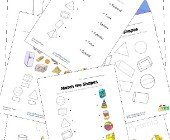## 3 Dimensional Shapes Worksheets

Check out our collection of 3D shapes worksheets f...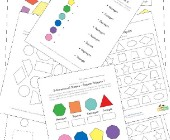## 2 Dimensional Shapes Worksheets

Check out our collection of 2D shapes worksheets f...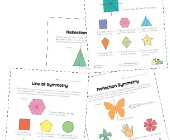## Symmetry Worksheets

Help kids learn about the concept of symmetry with...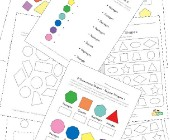## Geometry Worksheets

Help kids learn the early basics of geometry inclu...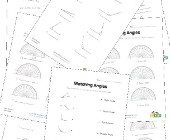## Angles Worksheets

Help kids learn about angles with this collection ...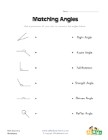## Matching Angles Worksheet

Draw a line to match each of the angles with the t...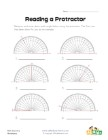## Reading a Protractor Worksheet 2

Read each protractor and write down the angles the...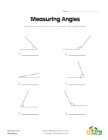## Measuring Angles Worksheet 1

Measure each of the angles with a protractor and w...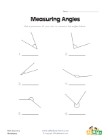## Measuring Angles Worksheet 2

Use a protractor to measure each of the angles.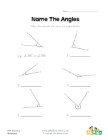## Name the Angles Worksheet

Follow the example and name each of the angles.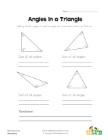## Angles in a Triangle Worksheet

Kids learn that the angles of a triangle always ad...